# First Steps Exploring the Google Cluster dataset with iPython

A lot of my research is based around developing and testing heuristic algorithms for various computation problems, in particular, I try to develop and adapt vector-packing based heuristics for scheduling virtual machines in distributed heterogeneous environments.

Toward this end, I've spent a fair amount of time investigating the large data trace released by google in 2011. The trace is available through Google Cloud Storage, and can be downloaded using the gsutil utility, which can be installed in a python virtual environment via pypi/pip. I want to be able to use this trace to generate synthetic problem instances to use to test my heuristics.

HUGOMORE42
In :
from os import listdir, chdir
print listdir('.')

['task_events', 'machine_events', 'README', 'machine_attributes', 'schema.csv', 'job_events', 'MD5SUM', 'runme.txt', 'task_constraints', 'task_usage', 'SHA1SUM', 'SHA256SUM']



The trace directory includes a number of sub-directories, each of which in turn is populated by some number of csv (comma separated values) files. These files have names like part-XXXXX-of-YYYYY.csv.gz, so should really be considered part of a continuous data stream. The values contained in the files for each directory file are described in the documentation and the schema.csv file. In places where they conflict schema.csv takes precedence.

According to both the documentation and schema.csv, the task event csv files contain the fields 'time', 'missing info', 'job ID', 'task index', 'machine_id', 'event type', 'user', 'scheduling class', 'priority', 'cpu request', 'memory request', 'disk space request', 'different machines restriction'. The most important for my purposes are probably 'time', 'event type', 'cpu request', and 'memory request'. The 'job ID' and 'task index' fields are needed to keep track of the evolution of a given task over time. The 'disk space request' field sounds interesting, but note that there is no corresponding disk-space field for a particular machine. The 'different machines restriction' field looks interesting, but I'm going to ignore it for now.

The first thing to observe is that it's not possible using this schema to simply look up the characteristics of a single task, or group of tasks. Rather, it's necessary to simulate the execution of the trace from the beginning (timestamp = 0) to a particular moment in time, and then one can investigate the state of only the tasks that are currently executing at that moment in time.

Now, we know the content of the files is arranged in simple chronological order, and we know that the first moment in the trace occurs at timestamp 0, so to find the last moment we can simply look for the last event in the last file. The python data analysis library makes loading compressed csv files from disk and working with column-oriented data relatively simple.

In :
print sorted(listdir('task_events'))[-1]

part-00499-of-00500.csv.gz


In :
from pandas import read_csv
from os import path
'priority', 'cpu_requested', 'mem_requested', 'disk', 'restriction']


2506199602822



So, the last event timestamp is 250619902822.

Since the trace is very large, it would be difficult to analyse the whole thing at once. What I propose to do instead is to define a sample of moments from which to collect statistics, primarily requested utilization, as well as a single moment in time for which to capture all of the information available about running tasks at that moment for further analysis.

In :
from random import randint, sample, seed
seed(83)
sample_moments = sorted(sample(xrange(250619902823), 200))
snapshot_moment = randint(0, 250619902822)
print snapshot_moment

12831046088


In :
from collections import OrderedDict

from pandas import DataFrame

samples_dicts = OrderedDict([])
sample_moments_iterator = iter(sample_moments)
current_sample_moment = next(sample_moments_iterator)

# Not the most elegant code I've ever written...
%%time

if current_sample_moment is not None and event['time'] > current_sample_moment:
samples_dicts[current_sample_moment] = ({'time' : current_sample_moment,
try:
current_sample_moment = next(sample_moments_iterator)
except StopIteration:
current_sample_moment = None

if tasks_df is None and event['time'] > snapshot_moment:

if event['event_type'] in [0, 7, 8]:
'machine_id' : event['machine_id'],
'cpu_requested' : event['cpu_requested'],
'mem_requested' : event['mem_requested']}
elif event['event_type'] in [2, 3, 4, 5, 6]:

if tasks_df is not None and current_sample_moment is None:
break

samples_df = DataFrame(samples_dicts.values())

print
print samples_df
print

<class 'pandas.core.frame.DataFrame'>
Int64Index: 147212 entries, 0 to 147211
Data columns (total 4 columns):
cpu_requested    146937  non-null values
machine_id       85256  non-null values
mem_requested    146937  non-null values
dtypes: float64(3), object(1)

<class 'pandas.core.frame.DataFrame'>
Int64Index: 200 entries, 0 to 199
Data columns (total 3 columns):
cpu_requested    200  non-null values
mem_requested    200  non-null values
time             200  non-null values
dtypes: float64(2), int64(1)

CPU times: user 6min 45s, sys: 3.94 s, total: 6min 49s
Wall time: 7min 41s



Whew! That took a while to load...

Now we can do some plotting of cpu and memory requested with matplotlib.

In :
import matplotlib.pyplot as plt

%%time
fig = plt.figure()
ax.plot(samples_df['time'], samples_df['cpu_requested'], label='cpu requested')
ax.plot(samples_df['time'], samples_df['mem_requested'], label='mem requested')
plt.xlim(min(samples_df['time']), max(samples_df['time']))
plt.legend()
plt.show()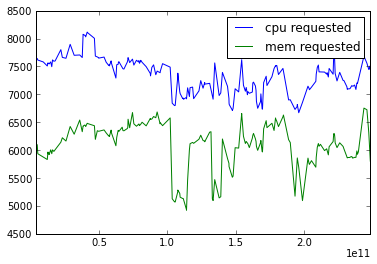CPU times: user 232 ms, sys: 4 ms, total: 236 ms
Wall time: 924 ms



We can use the same technique to collect information about machine events. From the documentation, or by looking in the 'schema.csv' file, we know that the csv files in this directory have five fields: time, machine ID, event type, platform ID, cpu capacity, and memory capacity. The event type is an integer, 0, 1, 2, with values meaning that a machine has been ADDED (0), REMOVED (1), or UPDATED (2). Fortunately, this directory only contains 1 file, rather than 500, so the code runs much more quickly this time around:

In :
machines_dict = {}
sample_moments_iterator = iter(sample_moments)
current_sample_moment = next(sample_moments_iterator)
machines_df = None

machine_events_csv_colnames=['time', 'machine_id', 'event_type', 'platform_id', 'cpu', 'mem']

%%time
for fn in sorted(listdir('machine_events')):
fp = path.join('machine_events', fn)
for index, event in machine_events_df.iterrows():

if current_sample_moment is not None and event['time'] > current_sample_moment:
tmp_machines_df = DataFrame(machines_dict.values())
samples_dicts[current_sample_moment].update({'cpu_available' : sum(tmp_machines_df['cpu']),
'mem_available' : sum(tmp_machines_df['mem'])})
try:
current_sample_moment = next(sample_moments_iterator)
except StopIteration:
current_sample_moment = None

if machines_df is None and event['time'] > snapshot_moment:
machines_df = DataFrame(machines_dict.values())

if event['event_type'] in [0, 2]:
machines_dict[event['machine_id']] = {'machine_id' : event['machine_id'],
'cpu' : event['cpu'], 'mem' : event['mem']}
elif event['event_type'] in :
del machines_dict[event['machine_id']]

if machines_df is not None and current_sample_moment is None:
break

machines_df = DataFrame(machines_dict.values())
samples_df = DataFrame(samples_dicts.values())

print machines_df
print
print samples_df
print

<class 'pandas.core.frame.DataFrame'>
Int64Index: 12486 entries, 0 to 12485
Data columns (total 3 columns):
cpu           12486  non-null values
machine_id    12486  non-null values
mem           12486  non-null values
dtypes: float64(2), int64(1)

<class 'pandas.core.frame.DataFrame'>
Int64Index: 200 entries, 0 to 199
Data columns (total 5 columns):
cpu_available    200  non-null values
cpu_requested    200  non-null values
mem_available    200  non-null values
mem_requested    200  non-null values
time             200  non-null values
dtypes: float64(4), int64(1)

CPU times: user 2.92 s, sys: 12 ms, total: 2.93 s
Wall time: 3.34 s



Okay, that's much more reasonable. Now we can graph how much was requested vs how much was available:

In :
%%time
fig = plt.figure()
ax.plot(samples_df['time'], samples_df['cpu_requested'], label='cpu requested')
ax.plot(samples_df['time'], samples_df['cpu_available'], label='cpu available')
ax.plot(samples_df['time'], samples_df['mem_requested'], label='mem requested')
ax.plot(samples_df['time'], samples_df['mem_available'], label='mem available')
plt.xlim(min(samples_df['time']), max(samples_df['time']))
plt.legend()
plt.show()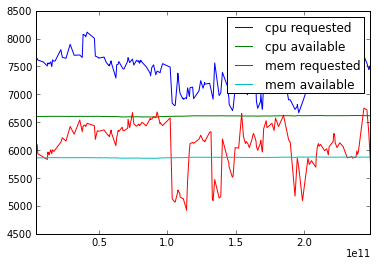CPU times: user 260 ms, sys: 0 ns, total: 260 ms
Wall time: 258 ms



So, while demand varies up and down among tasks that have been submitted to the system, availablity remains fairly constant. Demand for memory and cpu stay above availability fairly consistently, with some notable dips for memory.

An important question to ask is how well the scheduler used by google, which is based on a "cost" model, does at allocating resources. To find this out, we can look at the files in the 'task_usage' directory. These files have a slightly different schema, since they represent measurements taken at intervals by monitoring software. Each line has the fields 'start time', 'end time', 'job id', 'task index', 'machine id', 'mean CPU usage', 'canonical memory usage', 'assigned memory', 'unmapped page cache memory usage', 'page cache memory usage', 'maximum memory usage', 'mean disk I/O time', 'maximum disk space used', 'max CPU usage', 'max disk I/O time', 'cycles per instruction', 'memory accesses per instruction', 'sampling rate', and 'aggregation type'. The documentation suggests using the 'mean CPU usage' and 'canonical memory usage' for any modeling, and so these are the values that we will primarily be interested in.

Since the observations in this file can potentially overlap, rather than occurring in a strictly ordered sequence in time, we need to adopt a slightly different technique to read in the data.

In :
task_usage_csv_colnames=['starttime', 'endtime', 'job_id', 'task_idx', 'machine_id', 'cpu_usage', 'mem_usage',
'assigned_mem', 'unmapped_cache_usage', 'page_cache_usage', 'max_mem_usage', 'disk_io_time',
'max_disk_space', 'max_cpu_usage', 'max_disk_io_time', 'cpi', 'mai', 'sampling_rate', 'agg_type']

for moment in samples_dicts:
samples_dicts[moment].update({'cpu_usage' : 0.0, 'mem_usage' : 0.0})

tasks_dict[task].update({'cpu_usage' : 0.0, 'mem_usage' : 0.0, 'in_events' : True, 'in_usage' : False})

%%time

if laststart > max(sample_moments) and laststart > snapshot_moment:
break

for moment in samples_dicts:

'in_events': True, 'in_usage' : True})
else:
'cpu_usage' : usage['cpu_usage'], 'mem_usage' : usage['mem_usage'],
'in_events' : False, 'in_usage' : True}

samples_df = DataFrame(samples_dicts.values())
print samples_df

<class 'pandas.core.frame.DataFrame'>
Int64Index: 200 entries, 0 to 199
Data columns (total 7 columns):
cpu_available    200  non-null values
cpu_requested    200  non-null values
cpu_usage        200  non-null values
mem_available    200  non-null values
mem_requested    200  non-null values
mem_usage        200  non-null values
time             200  non-null values
dtypes: float64(6), int64(1)
<class 'pandas.core.frame.DataFrame'>
Int64Index: 208343 entries, 0 to 208342
Data columns (total 8 columns):
cpu_requested    208246  non-null values
cpu_usage        208343  non-null values
in_events        208343  non-null values
in_usage         208343  non-null values
machine_id       45939  non-null values
mem_requested    208246  non-null values
mem_usage        208343  non-null values
dtypes: bool(2), float64(5), object(1)
CPU times: user 11min 41s, sys: 38.8 s, total: 12min 20s
Wall time: 12min 38s



Now we can put demand, availability, and actual utilization on the same graphs. For the sake of clarity, we'll do a separate graph for each of cpu and memory.

In :
%%time
fig = plt.figure()
ax.plot(samples_df['time'], samples_df['cpu_requested'], label='cpu requested')
ax.plot(samples_df['time'], samples_df['cpu_available'], label='cpu available')
ax.plot(samples_df['time'], samples_df['cpu_usage'], label='cpu usage')
plt.xlim(min(samples_df['time']), max(samples_df['time']))
plt.legend()
plt.show()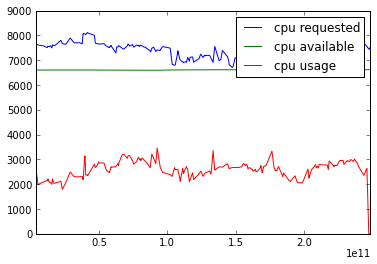CPU times: user 236 ms, sys: 0 ns, total: 236 ms
Wall time: 453 ms


In :
%%time
fig = plt.figure()
ax.plot(samples_df['time'], samples_df['mem_requested'], label='mem requested')
ax.plot(samples_df['time'], samples_df['mem_available'], label='mem available')
ax.plot(samples_df['time'], samples_df['mem_usage'], label='mem usage')
plt.xlim(min(samples_df['time']), max(samples_df['time']))
plt.legend()
plt.show()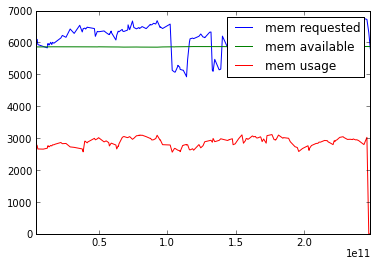CPU times: user 228 ms, sys: 0 ns, total: 228 ms
Wall time: 224 ms



In :
%%time
demand_pct = [100.0 * cpur / cpua for cpur, cpua in zip(samples_df['cpu_requested'], samples_df['cpu_available'])]
usage_pct = [100.0 * cpuu / cpua for cpuu, cpua in zip(samples_df['cpu_usage'], samples_df['cpu_available'])]
fig = plt.figure()
ax.plot(samples_df['time'], demand_pct, label='cpu % demand')
ax.plot(samples_df['time'], usage_pct, label='cpu % usage')
plt.xlim(min(samples_df['time']), max(samples_df['time']))
plt.legend()
plt.show()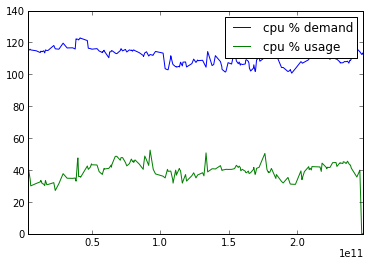CPU times: user 208 ms, sys: 4 ms, total: 212 ms
Wall time: 314 ms


In :
%%time
demand_pct = [100.0 * memr / mema for memr, mema in zip(samples_df['mem_requested'], samples_df['mem_available'])]
usage_pct = [100.0 * memu / mema for memu, mema in zip(samples_df['mem_usage'], samples_df['mem_available'])]
fig = plt.figure()
ax.plot(samples_df['time'], demand_pct, label='mem % demand')
ax.plot(samples_df['time'], usage_pct, label='mem % usage')
plt.xlim(min(samples_df['time']), max(samples_df['time']))
plt.legend()
plt.show()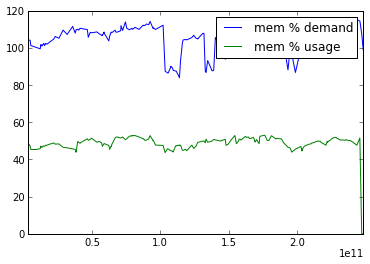CPU times: user 212 ms, sys: 0 ns, total: 212 ms
Wall time: 210 ms



So, while demand for cpu and memory tends to hang out around slightly over 100%, actual usage tends to hover closer to 40%--slightly under for CPU and slightly over for memory. I suppose you could argue that memory usage hovers closer to 50%, but still, it seems like a majority of both resources available is remaining unused. This observation seems consistent with the findings in the technical report by Reiss et al..

Now, there are some legitimate questions as to weather or not this is an appropriate trace to use to develop a model of "typical" cloud workloads--the tasks processed by google may be strongly idiosyncratic for any number of reasons--, but it does seem to be the best thing we have available for the moment. There are more mundane problems with the trace as well...clearly some of the data is missing or, in the case of utilization, inaccurate. For example, there are tasks present in the usage tables that are not present in the events tables, and vice versa.

In :
print tasks_df

<class 'pandas.core.frame.DataFrame'>
Int64Index: 208343 entries, 0 to 208342
Data columns (total 8 columns):
cpu_requested    208246  non-null values
cpu_usage        208343  non-null values
in_events        208343  non-null values
in_usage         208343  non-null values
machine_id       45939  non-null values
mem_requested    208246  non-null values
mem_usage        208343  non-null values
dtypes: bool(2), float64(5), object(1)
neither events nor usage: 0
usage, but not events: 56632
events, but not usage: 74018
both usage and events: 77693



Also, a lot of the actual request or usage data that is present seems inaccurate or misleading in some way, usually since missing or unspecified values may show up as 0.

In :
tasks_df[tasks_df['in_events'] == True]['cpu_requested'].hist()

Out:
<matplotlib.axes.AxesSubplot at 0x1cbc9c50>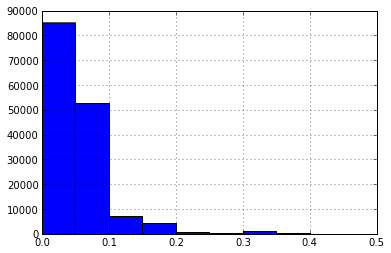In :
tasks_df[tasks_df['in_events'] == True]['mem_requested'].hist()

Out:
<matplotlib.axes.AxesSubplot at 0x746cdd0>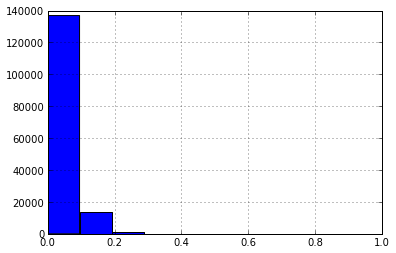In :
tasks_df[tasks_df['in_usage'] == True]['cpu_usage'].hist()

Out:
<matplotlib.axes.AxesSubplot at 0x125cd0d0>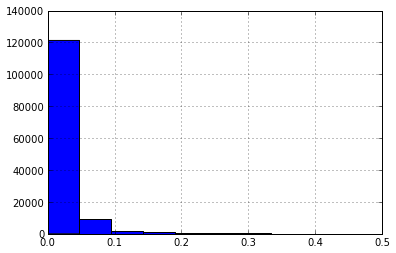In :
tasks_df[tasks_df['in_usage'] == True]['mem_usage'].hist()

Out:
<matplotlib.axes.AxesSubplot at 0xd6cef50>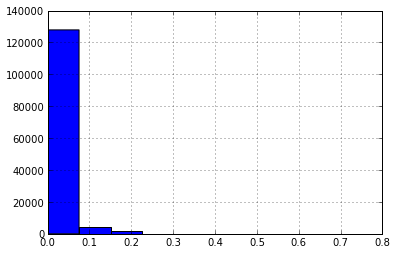Now, the using the usage data for task modeling is problematic for a few reasons beyond the fact that some of it may be missing or inaccurate. Since the usage of a given task depends non only on the characteristics of that task itself, but also on the performance of other tasks in the same job--since they may depend on one another--, and on the resource usage of other tasks scheduled to the same machine.

Resources requested isn't ideal either--user estimates for required resources are generally inaccurate--but it's a place to start. We can also filter out all of the "zero" requests, since these wouldn't affect vector packing algorithms directly anyway.

In :
tasks_nonzero_df = tasks_df[(tasks_df['cpu_requested'] > 0.0) & (tasks_df['mem_requested'] > 0.0)]

<class 'pandas.core.frame.DataFrame'>
Int64Index: 141697 entries, 1 to 208342
Data columns (total 8 columns):
cpu_requested    141697  non-null values
cpu_usage        141697  non-null values
in_events        141697  non-null values
in_usage         141697  non-null values
machine_id       45668  non-null values
mem_requested    141697  non-null values
mem_usage        141697  non-null values
dtypes: bool(2), float64(5), object(1)


In :
tasks_nonzero_df['cpu_requested'].hist()

Out:
<matplotlib.axes.AxesSubplot at 0x2ba76650>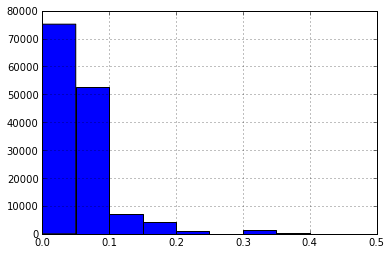In :
tasks_nonzero_df['mem_requested'].hist()

Out:
<matplotlib.axes.AxesSubplot at 0x2ba89110>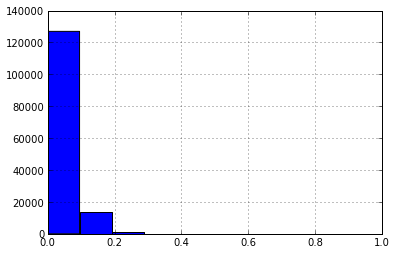In :
print min(tasks_nonzero_df['cpu_requested']), max(tasks_nonzero_df['cpu_requested'])

0.0006247 0.5
1.526e-05 0.9551



Even given the nonzero requirement, it seems like a majority of tasks appear 'very small'.

Machine characteristics look a little more like what we might expect.

In :
print machines_df['cpu'].hist()

Axes(0.125,0.125;0.775x0.775)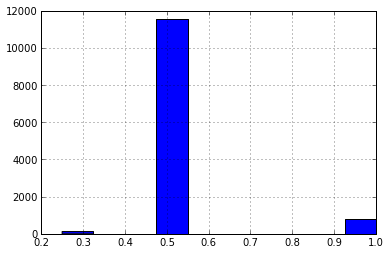In :
print machines_df['mem'].hist()

Axes(0.125,0.125;0.775x0.775)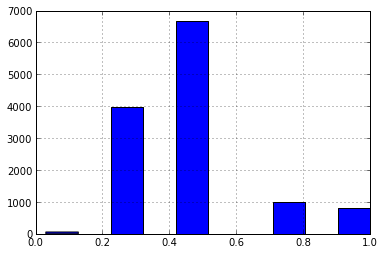In :
print min(machines_df['cpu']), max(machines_df['cpu'])
print min(machines_df['mem']), max(machines_df['mem'])

0.25 1.0
0.03085 1.0



It's interesting to consider the apparent density of tasks--that is, the task/machine ratio.

In :
print len(tasks_nonzero_df), len(machines_df), float(len(tasks_nonzero_df)) / len(machines_df)

141697 12486 11.3484702867



Even when removing all of the tasks with zero cpu or memory requrements the density remains over 11 tasks per machine.

Most of the researchers who have studied the trace have concluded that the distribution of task resource requirements is not easy to model statistically, and have suggested sampling as a way of deriving synthetic datasets. So, what I plan on doing is, for each synthetic problem set selecting a random moment in time, building up a collection of the currently running tasks and currently available machines, filtering out the tasks with zero requirements for cpu/memory, and then sampling from this data set to get a vector packing problem with a density of 12 tasks/machine. I'll try out some basic packing algorithms, see how they perform, and then proceed from there. One thing all of this tinkering with the dataset has made clear is that the currently available data is not ideal, and that the community would really benefit from more systematic collection of this type of data.##### Mark Stillwell
###### Site Reliability Engineer

Computer Scientist and Site Reliability Engineer, living in London